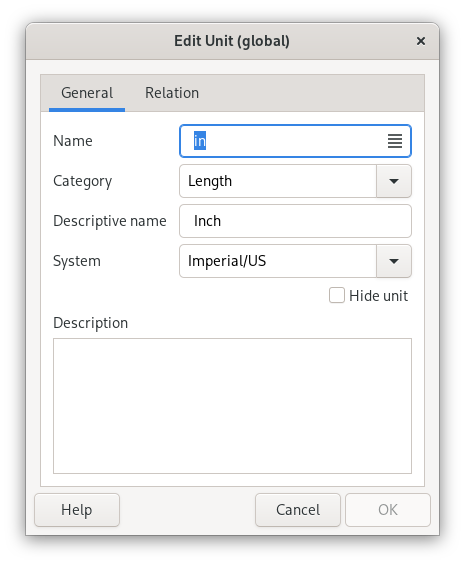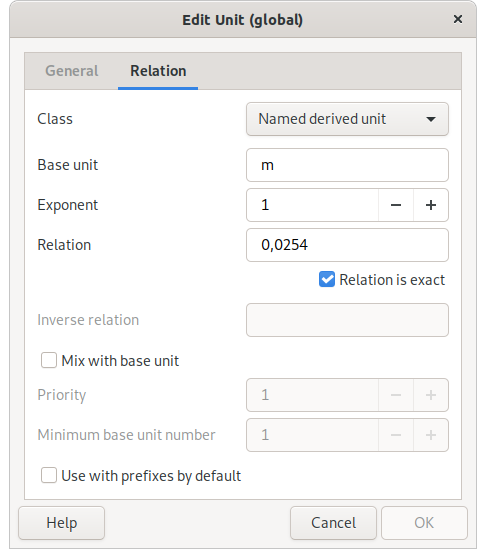# Chapter 9. Units

Currency
Conversion
Unit creation/editing

Units give numbers meaning in the real world. They specify what is measured by the numbers and the scale used. Qalculate! fully incorporates units in calculations and includes all standard SI units as well as many non-standard local units. For a complete list of available units see Appendix C, Unit List or the unit manager.

## Currency

Among units, Qalculate! has support for currencies with up-to-date exchange rates. Currencies are normally referenced with the standard three letter code due to name clashes, but a number of currency unit can also be accessed through their regular name and symbol. U.S. dollars can, for example, be referenced both as USD and dollar/dollars or the \$ symbol, unless the same name and/or symbol are used by the local currency.

The exchange rates can to be updated manually using FileUpdate Exchange Rates, or automatically at specific intervals (by default once every week, but this can be changed in the preferences dialog), when needed (when currencies are converted).

## Conversion

Expressions can be converted to a specific unit directly in the expression entry with the to operator (right arrows, including -> are also supported), which converts the left-hand expression (or the previous result) to a specified unit (e.g. 5 feet + 2 inches to cm converts the result of 5 feet + 2 inches to centimeters). Unit expressions may contain units, prefixes, exponents, multiplication and division. By default, no prefix will be added to units typed without prefix, but this behavior can be modified by putting a question mark in front of the unit expression (6 561 ft to m ≈ 2000 m but 6 561 ft to ?m ≈ 2 km). Type to optimal to get optimal unit, to base for base units, or to mixed to force the use of mixed units (see below). The to operator can also be used for other types of result transformations (see the section called “The to (and where) operators”).

Alternatively the unit conversion view can be used. It is opened using Conversion, Ctrl+O, EditConvert To Unit Expression... or Ctrl+T (the last two options moves the focus to the unit expression entry). Enter a unit expression in the text entry and press Enter (or click ), or select a unit from the list. An appropriate unit category will automatically be selected from units in the current result. If is checked subsequent results will automatically be converted (if the conversion view is open), and if is checked the optimal prefix will be set for unit expressions without any prefix. A unit can be inserted directly into the expression entry from the list using middle click or the context menu.

Figure 9.1. Unit Conversion ViewThe the result context menu and the menu associated with the keypad button also provides a list of units for conversion.

The final way to convert the result to another unit is to use EditConvert To Unit menu or to press in the unit manager, which also provides quick conversion of a value between two selected units. EditSet Prefix can be used to select a prefix.

It is also possible to let Qalculate! automagically convert the result to appropriate units with EditConvert To Optimal Unit or EditConvert To Base Units (or the corresponding options in the result context menu). If instead the corresponding choice is selected from Mode+Unit Display (or the result context menu), each result will automatically be converted until the choice is deactivated (Mode+Unit DisplayNo Automatic Conversion).

By default (controlled by ModeUnit DisplayConvert To Mixed Units) certain units, such as time units and many imperial/U.S. customary units, are automatically converted to mixed units (e.g. 60.2 minutes = 1 hour to 12 seconds). When explicitly converting to a specific unit the integer value of the selected unit is preserved (1.51 h to min = 90 min + 36 s) and mixed units is not used if otherwise the unit would not be present in the result (6 in to ft = 0.5 ft). This behavior can be modified by prepending the unit with a plus or minus sign (e.g. 174 cm to +in ≈ 5 ft + 8,5 in, 1.51 h to -min = 90.6 min).

## Unit creation/editing

There are three different unit classes in Qalculate! — base units, named derived units, and (unnamed) derived units. Base units are standard units that form the basis for all other units. Meters and seconds are typical base units. Derived units are defined in relation to other units. Named derived units are defined in relation to a single other unit, with an optional exponent (e.g. hour is defined as a named derived unit that equals 60 minutes which in turn is defined in relation to seconds). Unnamed derived units are defined by a unit expression with one or multiple units (e.g. J/s). They are primarily useful as basis for named derived units (e.g. W = J/s), and for simplified conversion and entry of the specified unit expression.

Select FileNewUnit, or click in the unit manager, and the unit edit dialog pops up.

Figure 9.2. Unit Edit Dialog (General)Base units and named derived units normally have three different name forms defined for use in expressions — abbreviation (e.g. m), singular (meter) and plural (meters). Unnamed derived units only have an internal name, which is used to reference the unit in definitions of other units, but which should not be used in mathematical expressions.

In addition category and descriptive name can be specified to keep the units well organized. A unit can be hidden from unit menus with the corresponding check box (this is primarily useful for some unnamed derived units which are only defined as basis for named derived units).

Depending on the unit class, different elements in the relation page of the dialog will be enabled.

Figure 9.3. Unit Edit Dialog (Relation)For named derived units, base unit, exponent and relation must all be specified (although the exponent and relation may both left as 1). The base unit can be of any unit class and it is recommended that named derived units are defined in relation to the closest unit (e.g. 1 ft = 3 hands, 1 hand = 4 in, and 1 in = 0.0254 m). The relation is usually just a number that tells how large quantity of the base unit is needed to get the derived unit (derived unit = relation × base unitexponent).

It is possible to create units with non-linear relation to the base unit. Replace the factor with \x and the exponent with \y (e.g. \x + 273.15 for degrees Celsius with Kelvin as base unit). For non-linear relations the reverse relation (for conversion back from the base unit) should also be specified (\x - 273.15 for degrees Celsius).

Base unit mixing can be enabled (by default) for named derived units. This is used for units such as feet and minutes, which are often combined with other units instead of using decimals (e.g. 5.25 ft = 5 ft + 3 in, 250 s = 4 min + 10 s). This behaviour can be fine-tuned used the priority and minumum base unit number properties.

For unnamed derived units a unit expression, with one or multiple units, must be specified in the base units field. This expressions may only contain units, prefixes, exponents, multiplication and division (e.g. km/h).